# Lecture 9 - Statistical Mechanics (2/11/99)Atomic Spectra --- | --- Radiative Transfer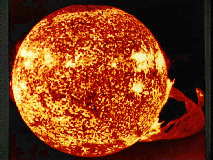Reading:
Chapter 8-4, 8-7 (ZG4)

Notes:
pages 34 - 38

The Sun and prominence from NASA's Skylab space station on December 19, 1973. (Courtesy NASA)
Key Question: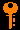## Investigations:

1. States - The Boltzmann Distribution
• How does the equilibrium between excitation and de-excitation determine the relative populations in different atomic energy states?
• What are the electronic energy levels in an atom?
• What is the root-mean-square kinetic energy per particle for a gas at temperature T?
• What is the expected ratio between numbers of atoms in two atomic energy states?
• What is the significance of the Boltzmann factor e-E/kT
• What is the mulitiplicity or statistical weight g of an energy level? How does that effect the populations?
• Where does the Boltzmann factor come from, and how is it related to the central limit theorem?
• What is statistical physics?
• At what temperature T is the Boltzmann factor on e-fold down for E = 1 eV?
• Where else does the Boltzmann factor apply?
2. Ionization - The Saha Equation
• What is ionization equilibrium?
• What do the ionization states I, II, III, etc signify?
• What are the ionization states of hydrogen? Helium?
• What is the ionization potential of hydrogen?
• How does the Boltzmann factor affect the ionization?
• How does the ionization depend upon the electron density?
• What is the statistical weight factors for ionization?
• What is the Saha equation?
• Do you expect the solar photosphere to be ionized?
• How do you compute the fractional ionization of a gas?
• What is the electron pressure in the solar photosphere?
• What is the expected fractional ionization of the solar photosphere?
3. The Balmer Series - Boltzmann + Saha Equations
• What is the Balmer series in hydrogen?
• On the population of which level does the Balmer series line strength depend?
• At what temperature should the Balmer series be the strongest?
• Why does the Balmer series fade at high and low temperatures?
• How can you use line strengths to find conditions in an atmosphere?
4. The Solar Photosphere
• If the Sun is just a ball of hot gas, why does it appear to have a distinct surface?
• What is above and below the surface of the Sun? What keeps them apart?
• What do we mean by the photosphere?
• What do we see when we look at the photosphere of the Sun?
5. Radiative Transfer I - The Problem
• What can happen to photons on their way through a medium?
• Why do we need only consider absorption and emission, and not scattering separately?
• What do we mean by a "beam" of photons?

## Ionization States

The process of ionization and recombination and the ionization states of hydrogen and helium: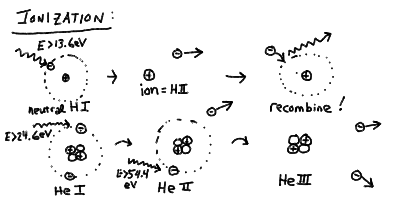## The Boltzmann Equation

The ratio of the number density (in atoms per m^3) of atoms in energy state B to those in energy state A is given by

NB / NA = ( gB / gA ) exp[ -(EB-EA/kT ]

where the g's are the statisical weights of each level (the number of states of that energy). Note for the energy levels of hydrogen

gn = 2 n2

which is just the number of different spin and angular momentum states that have energy En.

## The Saha Equation

The textbook is a little confusing on this subject, as they just give in (8-31)

Ni+1 / Ni = A Ne-1 (kT)3/2 exp[ -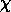i/kT ]

but don't say what A is! In class, we gave the full equation

Ni+1 / Ni = ( 2Qi+1 / NeQi ) ( 2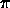mekT / h2 ) exp[ -i/kT ]

where Q is the partition function for the two ionization states (normalized to the ground state)

Q = g1 +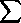n=2 gn exp[ En - E1 / kT ]

which numerically becomes

Ni+1 / Ni = ( 2.41 x 1021 m-3 / Ne ) ( 2Qi+1 / Qi ) T3/2 exp[ -i/kT ]

(note that for HII Q=1 and HI Q=2 so the partition funtion terms go to unity). We also used the fact that the electrons can be seen to form a gas

Pe = Ne k T

so we can write the Saha equation as

Ni+1 / Ni = ( 3.33 x 10-2 Pa / Pe ) ( 2Qi+1 / Qi ) T5/2 exp[ -i/kT ]

since the density and temperatures vary through most stellar atmospheres but the are roughly isobaric.

Note that the Saha equation is just an application of the Boltzmann equation to the case of ionization states! If you are trying to compute the occupancy of different energy states in the same ionization state (be H I, He I, He II, etc) then you need to use the Boltzmann equation. If you are trying to compute the relative fraction of atoms in one particular energy state of one particular ionization state to the total number in all energy and ionization states, then you need to use both equations!

For example, to calculate the fraction in the n=2 state of H_I to the total number density N, which can be either in the ionization state H I (any n) or H II. Since we found in class (and you can demonstrate easiy) that almost all of the H I atoms are in the n=1 ground state, N_1 ~ N_I, and thus

N2/N = ( N2/N1 ) / ( NI/N1 + NII/N1 )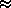( N2/N2 / ( 1 + NII/NI )

on which you can use the Boltzmann equation (for N_2/N_1) and the Saha equation (for N_II/N_I).

## Types of Spectra: Continuum, Emission, Absorption

Three types of spectra: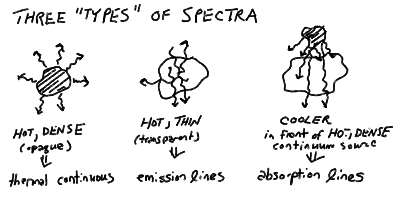## More Atomic Spectra in Outline:

1. The Bohr Atom: Orbital Radii and Energy Levels
• For an electron wave not to interfere with itself, there must be an integer number n wavelengths on an orbit. The smallest allowed orbit contains a single wavelength.
• The radius of the orbit is:

r = (h/2Pi)2 n2 / (m e2)

(where we absorb the Coulomb constant into e).

a0 = h2 / (4 Pi2 m e2)

• Then,

r = a0 n2

• The energy of a moving particle under a force is:

E = (m v2 / 2) - (e2 / r)

• The first term (m v^2/ 2) is the kinetic energy, and the second term (-e^2/r) is the potential energy.
• Using the Coulomb and centripetal forces, we find:

E = - e2/2r = -e2 / (2 a_0 n2)

• Thus, E = - E_R / n^2 where E_R = e^2 / (2 a_0) is the Rydberg Energy for hydrogen.
• Thus, the energy levels are spaced like the inverse square of the level number n, while the radii are spaced like the square of n.
• From all the constants you can compute the values of the Bohr Radius a_0 = 5.29 x 10^-11 m = 0.053 nm, and the Rydberg energy E_R = 2.18 x 10^-18 Joules = 13.6 eV.
• We define the the electron volt (eV) as the energy gained by an electron accelerated through a voltage difference of 1 volt (like from a battery).

1 eV = 1.602 x 10-19 J

• An electron at rest infinitely far away from the proton (n = infinity) has E = 0, while the ground state n = 1 has an energy E = -13.6 eV.
• It takes an energy of 13.6 eV to remove an electron in the ground state from the hydrogen atom (ionization, see below).
2. Hydrogen Energy Levels and Line Series
• Now that we have the energy levels of hydrogen, we can compute easily the wavelengths of light emitted and absorbed on transitions between different orbits.
• These are called spectral lines because in the spectrum of a star (the brightness versus frequency) these show up as narrow "lines" at specific wavelengths.
• The energy of a photon of wavelength L is just E = h c / L. The energy difference between a lower level m and an upper level n > m is just

( En - Em ) = ER ( 1/m2 - 1/n2 )

• Thus, the wavelength L corresponding to this difference is given by:

(h c / Lmn) = ( En - Em )

• If we define the Rydberg wavelength L_R = (h c / E_R) = 91.18 nm, then:

LR / Lmn = 1/m2 - 1/n2

• We see immediately that the largest energy transition is from the ground state m=1 to n=infinity (13.6 eV) has a wavelength of 91.18 nm. Photons of this wavelength or shorter will remove the electron from hydrogen entirely (see Ionization below). All other hydrogen lines have wavelengths longer than this.
• There are series of lines corresponding to transitions between each lower level m and all upper levels. The series for the ground state m=1 and all higher levels is called the Lyman Series. The longest Lyman line corresponds to an upper level n=2, and from our formula can be seen to have a wavelength of 121.6 nm. All the other Lyman lines have wavelength between this and the Lyman limit of 91.18 nm.
• These series of lines have a charateristic spacing that converge closer and closer to the limit for n=infinity.
• The longest wavelength line in a given series is designated by the Greek letter Alpha. The next is Beta, then Gamma, then Delta, and so on. The Lyman series is thus Ly Alpha (121.6 nm), Ly Beta (102.6 nm), Ly Gamma (97.25 nm), and so on. We abbreviate Lyman as Ly.
• From those wavelengths, you can see that the Lyman line series is hard to see since it is in the ultraviolet part of the spectrum which is absorbed by the Earth's atmosphere. It is much easier to see the next series corresponding to a lower level m=2.
• The Balmer Series is between a lower level m=2 and those above. The first line is H Alpha (the designation for Balmer for historical reasons is H for Hydrogen) at 656.6 nm, with H Beta (486.3 nm), H Gamma (434.3 nm), H Delta (410.3 nm) and so on up to the Balmer limit of 364.7 nm.
• The Balmer lines are in the visible part of the spectrum and are prominent in most stars.
• There are other series of lines, Paschen for m=3 ( Pa Alpha = 1876 nm, limit = 820.6 nm) and Brackett for m=4 ( Br Alpha = 4052 nm, limit = 1459 nm ). These lines are in the infrared part of the spectrum and harder to measure from the ground.
• As is common in this subject, astronomers are not content to use normal units for wavelength like nanometers. They use the Angstrom (A) which is just one-tenth of a nm, or 1 A = 10^-10 m, and so 10 A = 1 nm. Thus, the wavelength of H Alpha is 6565 A, Ly Alpha is 1216 A, and the Lyman limit is 912 A.
• The Bohr radius a_0 is 0.529 A. Most atoms are a few Angstroms across.
3. Ionization
• If a photon of short enough wavelength (high energy) is absorbed, for example shorter than 91.18 nm for hydrogen, then the electron is knocked clear out of the atom, and it is free to roam the universe at will.
• This process is called ionization and can also be caused by a strong enough collision. Ionization leaves behind a free electron, and an atom that is now positively charged - and is called an ion.
• The free electron can later (actually not very much later ususally) recombine with this or another ion, since it is attracted by the electric force.
• In multi-electron atoms, such as any other type of atom than hydrogen, it takes more energy to knock out successive electrons after you remove the first one. This is just because there is more charge pulling on fewer electrons (which tend to screen each other from the nucleus).
• Also, it takes more energy to remove electrons that are closer to the nucleus, since they have a more negative energy E. It is always the outermost, or the least tightly bound, electron that is first removed upon ionization.
• Ionization states of atoms are designated by the chemical symbol for the element (H=hydrogen, He=Helium, C=Carbon, etc) and a Roman numeral (I=neutral, II=singly ionized, III=doubly ionized, etc.)
• Thus neutral hydrogen is H I, and ionized hydrogen (a proton and an electron) is H II.
• Neutral Helium is He I, singly ionized Helium is He II (one electron removed), and doubly ionized Helium is He III.
• It takes 13.6 eV to turn H I into H II. It takes 24.6 eV to turn He I into He II, and another 54.4 eV to turn He II into He III. The ionization energy of He 24.6 eV is slightly less than twice hydrogen because of the screening of the other electron. The ionization energy from He II to He III is four times 13.6 eV because the energy goes as the charge squared, and He II looks like hydrogen with a single electron, but more positive charge in the nucleus.
4. Electron Orbitals and Quantum Numbers
• We have established orbits, or electron shells, with increasing radius from the nucleus

r = a0 n2

and increasing energy

E = -ER / n2

In some sense, we have labeled these orbits or states by the magic number n, which increased from the ground state n = 1.
• A good question was asked in class - we have been drawing the electron orbit as a circle, like a planet's orbit. But atoms are spherical. Should we take that into account?
• The answer to that is yes - in fact, it is crucial for the structure of atoms and how atoms interact with each other. If orbits were circular, then we can see that we can only put one electron on a given shell without it interfering. It turns out if you compute the number of separate waves that you can put on a spherical shell at level n, you find that you can actually put n^2 waves and still be able to tell them apart! (This is like the surface area of the sphere goes like the radius squared.) Thus, you can put more than one electron into each shell, as long as you can tell them apart.
• The way you tell apart two electrons is by labeling them by a series of quantum numbers, like we tell apart houses by addresses. The level n is a quantum number.
• You might expect the waves on a given shell to be specified by two quantum numbers, like positions on the Earth are specified by two coordinates latitude and longitude, and stars on the sky by declination and right ascension. Since we have used the letter n for the radial quantum number, we will use the letters l and m for the two angular quantum numbers.
• The quantum number l is roughly equivalent to a polar angle coordinate like latitude and declination or elevation. It counts the number of waves from "pole" to "pole" of the shell.
• The quantum number m is similarly equivalent to an azimuthal angle coordinate like longitude, right ascension, or azimuth. It counts the number of waves around the "equator" of the shell.
• Note that when we put our waves onto the sphere, they no longer become sinusoids with n wavelengths around a circle. Since they have to match up around all directions on the sphere, they take on the properties of special functions called spherical harmonics. They can have differing numbers of waves in the two angular directions on the sphere - which is how we can tell them apart, and why there are n^2 different ones!
• For a given level n, the quantum number l can take on integer values from 0, 1, up to (n-1). There are thus n different ones of these.
• For a given value of l, there quantum number m can take on integer values from -l, -l+1, ..., 0, 1, ..., up to +l. There are (2l+1) different values for these.
• Thus, if you add it all up, there are n^2 different allowed combinations for l and m.
• It turns out that in additon to the radial and angular quantum numbers n,l,m. particles like electrons and protons have their own internal quantum number s, which stands for spin. In a crude sense, it is useful to think of particles as little spinning tops, with an axis of rotation. For electrons and protons, the quantum number s can take on one of two values of +1/2 or -1/2. These correspond to states with the spin pointing "downward" and the spin pointing "upward" (or clockwise or counterclockwise rotation) from some given point of view.
• If we take into account this extra electron quantum number, we see we can actually fit twice as many electrons into a shell as we could without it, and still tell them apart. Thus, there are places for 2n^2 electrons in each level n.
• The requirement that we be able to "distinguish" two electrons, and their wave-functions, from each other, thus requiring them to be labeled by distinct series of quantum numbers, is known as the Pauli Exclusion Principle. The upshot of this is you can only put 0, 1, or 2 electrons in any given n,l,m state, and if you put two, they must have different orientations of s.
• We call each different value of l, for a given level n, an electron orbital. The orbitals were historically given designations of s, p, d, f, etc. for values of l = 0, 1, 2, 3, etc. respectively. Thus, the orbital n=1, l = 0 would be called "1s", n=3, l = 2 is "3d", and so on.
• In quantum mechanics, the interpretation of the wave-function of a particle is that it represents the probability of where the particle can be found. Thus, the crests and troughs of the wave represent places where is is likely that the particle is, while the zero-points of the wave are places where the particle isn't. In the quantum mechanical picture of the Universe, you can never pinpoint exactly where a particle is - only give probabilities as given by the wave-function of that particle.
• Thus, the l and m quantum numbers tell where on the shell the electron wave is "localized".
• The s orbital (l=m=0) is a wave-function that is uniformly spread over the shell. You can place only two electrons in an s orbital, and they must have opposite spins s.
• The p orbital (l=1, m=-1,0,+1) is a single-wavelength wavefunction, so the electron probability is concentrated at either of two opposite ends. There are three different orientations, with the electron location concentrated along the x, y, or z axis (any three perpendicular directions). You can place up to 6 electrons in p orbitals, though if you put two in the same m sub-orbital, then they must be of opposite spins.
• The chemical properties of atoms are governed by the which orbitals are occupied in the outermost shells of electrons - the so called valence electrons, since these are the least tightly bound and are available for sharing with other atoms. The organization of the Periodic Table of Elements is entirely along the lines of the electron orbitals. Two atomic elements with their outermost electrons in similar orbitals (though in different n shells) will have the same chemical properties. Most of chemistry has its base in the physics of atomic structure!Prev Lecture ---Next Lecture ---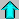Astr12 Index ---Astr12 Home

smyers@nrao.edu Steven T. Myers